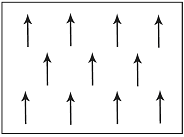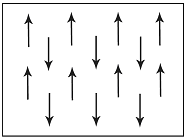# 29.11: Questions

$$\newcommand{\vecs}{\overset { \rightharpoonup} {\mathbf{#1}} }$$ $$\newcommand{\vecd}{\overset{-\!-\!\rightharpoonup}{\vphantom{a}\smash {#1}}}$$$$\newcommand{\id}{\mathrm{id}}$$ $$\newcommand{\Span}{\mathrm{span}}$$ $$\newcommand{\kernel}{\mathrm{null}\,}$$ $$\newcommand{\range}{\mathrm{range}\,}$$ $$\newcommand{\RealPart}{\mathrm{Re}}$$ $$\newcommand{\ImaginaryPart}{\mathrm{Im}}$$ $$\newcommand{\Argument}{\mathrm{Arg}}$$ $$\newcommand{\norm}{\| #1 \|}$$ $$\newcommand{\inner}{\langle #1, #2 \rangle}$$ $$\newcommand{\Span}{\mathrm{span}}$$ $$\newcommand{\id}{\mathrm{id}}$$ $$\newcommand{\Span}{\mathrm{span}}$$ $$\newcommand{\kernel}{\mathrm{null}\,}$$ $$\newcommand{\range}{\mathrm{range}\,}$$ $$\newcommand{\RealPart}{\mathrm{Re}}$$ $$\newcommand{\ImaginaryPart}{\mathrm{Im}}$$ $$\newcommand{\Argument}{\mathrm{Arg}}$$ $$\newcommand{\norm}{\| #1 \|}$$ $$\newcommand{\inner}{\langle #1, #2 \rangle}$$ $$\newcommand{\Span}{\mathrm{span}}$$$$\newcommand{\AA}{\unicode[.8,0]{x212B}}$$

## Quick questions

You should be able to answer these questions without too much difficulty after studying this TLP. If not, then you should go through it again!

Which picture best describes ferromagnetism?

 abcdB. In a ferromagnetic material below the Curie temperature all the dipole moments are aligned parallel to each other.

Which 3 elements are ferromagnetic at room temperature?

 a Mn, Cr, Fe b Mg, Ti, Ni c Fe, Ni, Co d Co, Ce, Mn

C. There are only 4 ferromagnetic elements at room temperature. Of these, iron (Fe), nickel (Ni), and cobalt (Co) are shown above. The fourth is gadolinium (Gd).

What happens to ferromagnetic materials above the Curie Temperature?

 a They become diamagnetic b They become gases c They become antiferromagnetic d They become paramagnetic

D. Above the Curie temperature there is a large enough contribution from the thermal energy to overcome the cooperative ordering of the magnetic dipole moments and so the material becomes paramagnetic.

What is the main driving force for the formation of domains?

 a Magnetostatic energy b Magnetocrystalline energy c Magnetostrictive energy d All of the above